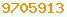# shift : Java Glossary

shift
Java has a number of operators for multiplying/dividing by powers of two, i.e. shifting left or right by bits. These are very fast operations, much faster than the using multiplication, division and Math.pow.
Shift Operators
Operation Operator Example Effect
left shift << 0x0a << 40xa0 Shift left n bits, multiply by 2n
logical right shift >>> 0xa0 >>> 40x0a
0xffffffa0 >>> 40x7ffffffa
unsigned shift right n bits, 0 bit fills in on the left, divide by 2n
arithmetic right shift >> 0xa0 >> 40x0a
0xffffffa0 >> 40xfffffffa
signed shift right n bits, sign bit fills in on the left, divide by 2n
left shift <<= x <<= 4 shorthand for x = x << 4
logical right shift >>>= x >>>= 4 shorthand for x = x >>> 4
arithmetic right shift >>= x >>= 4 shorthand for x = x >> 4

## Creating Masks Dynamically

You can create powers of two, (one-bit masks) by shifting 1 left a variable amount, e.g. (1<<n). Normally you create masks with hex literals, e.g. 0x007f. To dynamically create a mask with n low order 1’s, use (1<<n)-1. Beware, for int this will not work for n=32 since shifts are done modulo 32.

## Shiftless Shift

The value of the shift should be in the range 0..31. You can’t reverse the direction of the shift by using a negative count. If you use a number outside the range, it will be corralled back into the range 0..31 by taking a modulo 32. You would think that 1 << 66 would shift the one right off the left end and give you a result of 0, but int shifts are done modulo 32, so that is equivalent to 1 << 2 giving you a result of 4. Similarly, long shifts are done modulo 64 bits. This applies to <<<, << and >>. As a corollary, shifting left 32 bits does not clear an int. It is a no-op! Be especially careful when working with ints that will eventually be promoted.

Be especially careful with any calculated shift operands. The shift modulo behavior makes creation of bit selections masks unnecessarily tricky. Suppose you want a general way of extracting bits i through j of an int. An obvious way to do it is to right shift the input by j bits, then & it with a mask containing j-i+1 one bits at the low order end. An obvious way to construct an n-bit right justified mask is (1<<n)-1. This is not correct in Java for n==32. (1 <<32) is 1, not 0, so subtracting 1 from it will result in a zero mask rather than an all-ones mask.

Java works this way for speed since many CPUs (Central Processing Units) work that way.

Oracle RFE number 4495754 : Shift fixed for n==32

In the meantime here is a little class you can use:This page is postedon the web at: http://mindprod.com/jgloss/shift.html Optional Replicator mirror of mindprod.com on local hard disk J: J:\mindprod\jgloss\shift.htmlPlease read the feedback from other visitors, or send your own feedback about the site.Contact Roedy. Please feel free to link to this page without explicit permission. Canadian Mind Products IP:[65.110.21.43] Your face IP:[18.205.176.100] Feedback You are visitor number